# ISEE Lower Level Quantitative : Quadrilaterals

## Example Questions

### Example Question #21 : Plane Geometry

What is the perimeter of the polygon below?Possible Answers: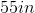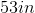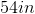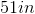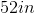Correct answer:Explanation:

To find the area of a perimeter, we add all of the side lengths together.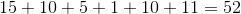### Example Question #22 : Plane Geometry

What is the perimeter of the polygon below?Possible Answers: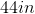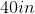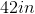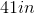Correct answer:Explanation:

To find the area of a perimeter, we add all of the side lengths together.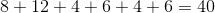### Example Question #23 : Plane Geometry

What is the perimeter of the polygon below?Possible Answers: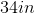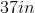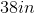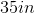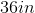Correct answer:Explanation:

To find the area of a perimeter, we add all of the side lengths together.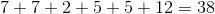### Example Question #24 : Plane Geometry

What is the perimeter of the polygon below?Possible Answers: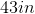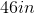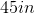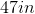Correct answer:Explanation:

To find the area of a perimeter, we add all of the side lengths together.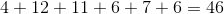### Example Question #25 : Plane Geometry

What is the perimeter of the polygon below?Possible Answers:Correct answer:Explanation:

To find the area of a perimeter, we add all of the side lengths together.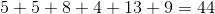### Example Question #26 : Plane Geometry

What is the perimeter of the polygon below?Possible Answers: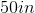Correct answer:Explanation:

To find the area of a perimeter, we add all of the side lengths together.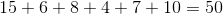### Example Question #27 : Plane Geometry

What is the perimeter of the polygon below?Possible Answers: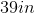Correct answer:Explanation:

To find the area of a perimeter, we add all of the side lengths together.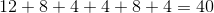### Example Question #28 : Plane Geometry

What is the perimeter of the polygon below?Possible Answers: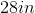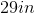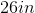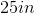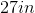Correct answer:Explanation:

To find the area of a perimeter, we add all of the side lengths together.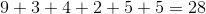### Example Question #29 : Plane Geometry

What is the perimeter of the polygon below?Possible Answers:Correct answer:Explanation:

To find the area of a perimeter, we add all of the side lengths together.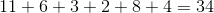### Example Question #30 : Plane Geometry

What is the perimeter of the polygon below?Possible Answers: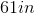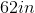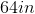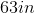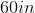Correct answer:Explanation:

To find the area of a perimeter, we add all of the side lengths together.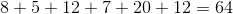### All ISEE Lower Level Quantitative Resources